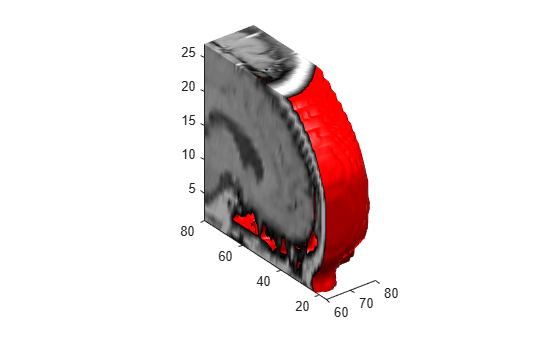subvolume

Extract subset of volume data set

Syntax

[Nx,Ny,Nz,Nv] = subvolume(X,Y,Z,V,limits)
[Nx,Ny,Nz,Nv] = subvolume(V,limits)
Nv = subvolume(...)

Description

[Nx,Ny,Nz,Nv] = subvolume(X,Y,Z,V,limits) extracts a subset of the volume data set V using the specified axis-aligned limits. limits = [xmin,xmax,ymin, ymax,zmin,zmax] (Any NaNs in the limits indicate that the volume should not be cropped along that axis.)

The arrays X, Y, and Z define the coordinates for the volume V. The subvolume is returned in NV and the coordinates of the subvolume are given in NX, NY, and NZ.

[Nx,Ny,Nz,Nv] = subvolume(V,limits) assumes the arrays X, Y, and Z are defined as

[X,Y,Z] = meshgrid(1:N,1:M,1:P)

where [M,N,P] = size(V).

Nv = subvolume(...) returns only the subvolume.

Examples

collapse all

Load the mri data set, which is a collection of MRI slices of a human skull. Then:

• Squeeze the four-dimensional array into three dimensions and extract a subset using subvolume.

• Use a patch to display an isosurface of the skull outline.

• Add a second patch with interpolated face colors.

• Set the view of the object.

• Change the colormap to a grayscale colormap.

• Add lights to the right and left of the camera to illuminate the object.

D = squeeze(D);
[x,y,z,D] = subvolume(D,[60,80,nan,80,nan,nan]);
p1 = patch(isosurface(x,y,z,D, 5),...
'FaceColor','red','EdgeColor','none');
isonormals(x,y,z,D,p1);
p2 = patch(isocaps(x,y,z,D, 5),...
'FaceColor','interp','EdgeColor','none');
view(3);
axis tight;
daspect([1 1 0.4])
colormap(gray(100))
camlight right;
camlight left;
lighting gouraud